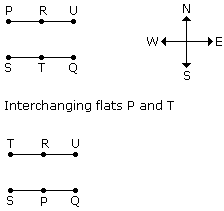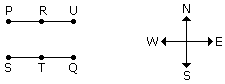Verbal Reasoning - Direction Sense Test

Exercise :: Direction Sense Test - Direction Sense 3

Each of the following questions is based on the following information:
1. Six flats on a floor in two rows facing North and South are allotted to P, Q, R, S, T and U.
2. Q gets a North facing flat and is not next to S.
3. S and U get diagonally opposite flats.
4. R next to U, gets a south facing flat and T gets North facing flat.
1.

If the flats of P and T are interchanged then whose flat will be next to that of U?

 A. P B. Q C. R D. T

Explanation:Hence flat R will be next to U.

2.

Which of the following combination get south facing flats?

 A. QTS B. UPT C. URP D. Data is inadequate

Explanation:Hence URP flat combination get south facing flats.

3.

The flats of which of the other pair than SU, is diagonally opposite to each other?

 A. QP B. QR C. PT D. TS

Explanation:Hence QP is diagonally opposite to each other.

4.

Whose flat is between Q and S?

 A. T B. U C. R D. P